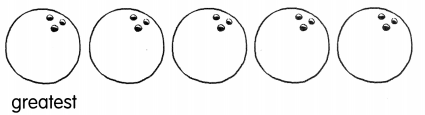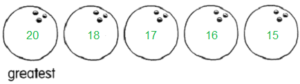# Math in Focus Grade 1 Chapter 7 Practice 4 Answer Key Making Patterns and Ordering Numbers

This handy Math in Focus Grade 1 Workbook Answer Key Chapter 7 Practice 4 Making Patterns and Ordering Numbers detailed solutions for the textbook questions.

## Math in Focus Grade 1 Chapter 7 Practice 4 Answer Key Making Patterns and Ordering Numbers

Solve.

Question 1.
Alex uses circles to make a pattern. How many circles come next in the pattern? Draw the circles in the empty box. Write the number of circles below this box.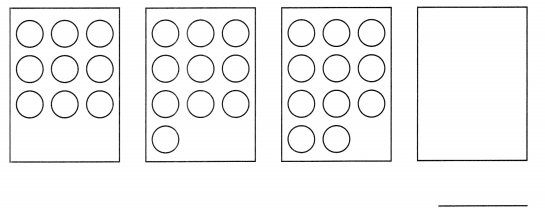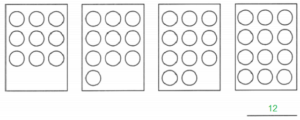Complete the patterns.

Question 2.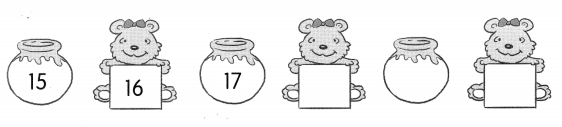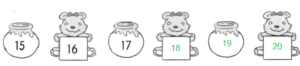Question 3.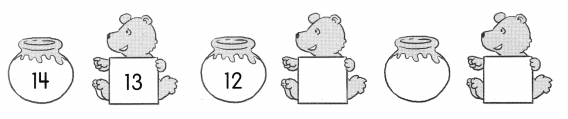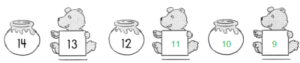Look at the numbers. Fill in the blanks.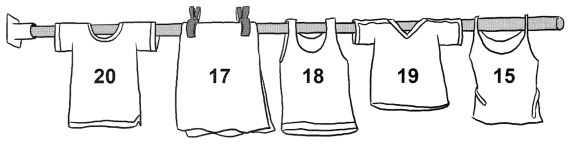Question 4.
________ is 2 more than 15.
Answer: 17 is 2 more than 15.

Question 5.
________ is 2 less than 20.
Answer: 18 is 2 less than 20.

Question 6.
1 more than 18 is ___.
Answer: 1 more than 18 is 19.

Question 7.
2 less than 19 is ____.
Answer: 2 less than 19 is 17

Complete the number patterns.

Question 8.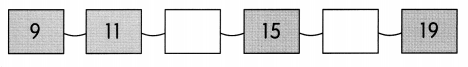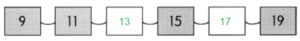Question 9.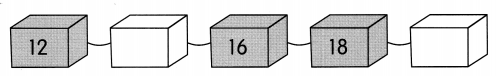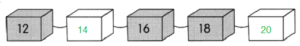Question 10.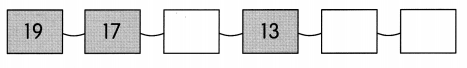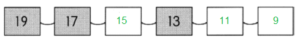Question 11.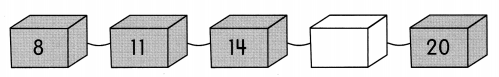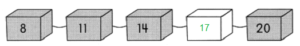Question 12.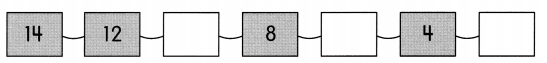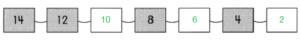Help Rosa order the bowling pins and balls.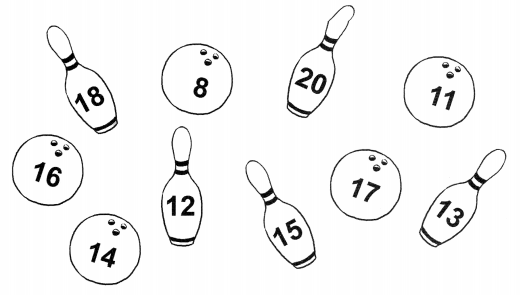Question 13.
Write the numbers on the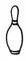in order from greatest to least.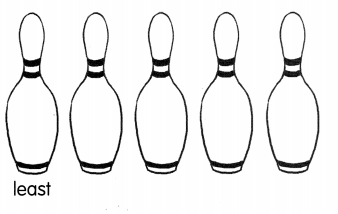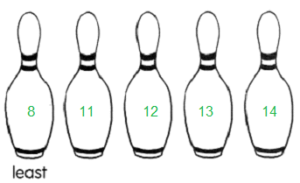Write the numbers on the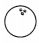in order from greatest to least.